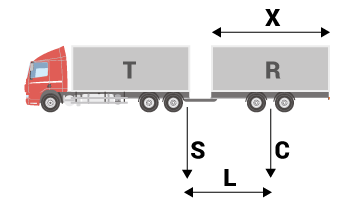## Steering axle trailersD-Value = g x
T x R / T + R

where:

• g = Gravitational acceleration; 9.81 m/s2;
• T = Technically admissible maximum weight of towing vehicle in tons;
• R = Technically admissible maximum weight of towing vehicle in tons;
• D-Value = Theoretical load for the determination of the theoretical horizontal dynamic force between towing vehicle and steering axle trailer;
T ton
R ton

D-Value kN

## Central axle trailersDc-Value = g x
T x C / T + C

where:

• g = Gravitational acceleration; 9.81 m/s2;
• T = Technically admissible maximum load of the towing vehicle in tons, including the static vertical load transmitted to the coupling by the drawbar eye;
• C = Sum of the maximum axle loads transmitted to the ground by ￼the trailer in tons.

T ton
R ton
S ton
C ton
X m
L m

T* ton
Dc-Value kN
V kN